# 概述

JDK 1.8对HashMap进行了比较大的优化，底层实现由之前的“数组+链表”改为“数组+链表+红黑树”，本文就HashMap的几个常用的重要方法和JDK 1.8之前的死循环问题展开学习讨论。JDK 1.8的HashMap的数据结构如下图所示，当链表节点较少时仍然是以链表存在，当链表节点较多时（大于8）会转为红黑树。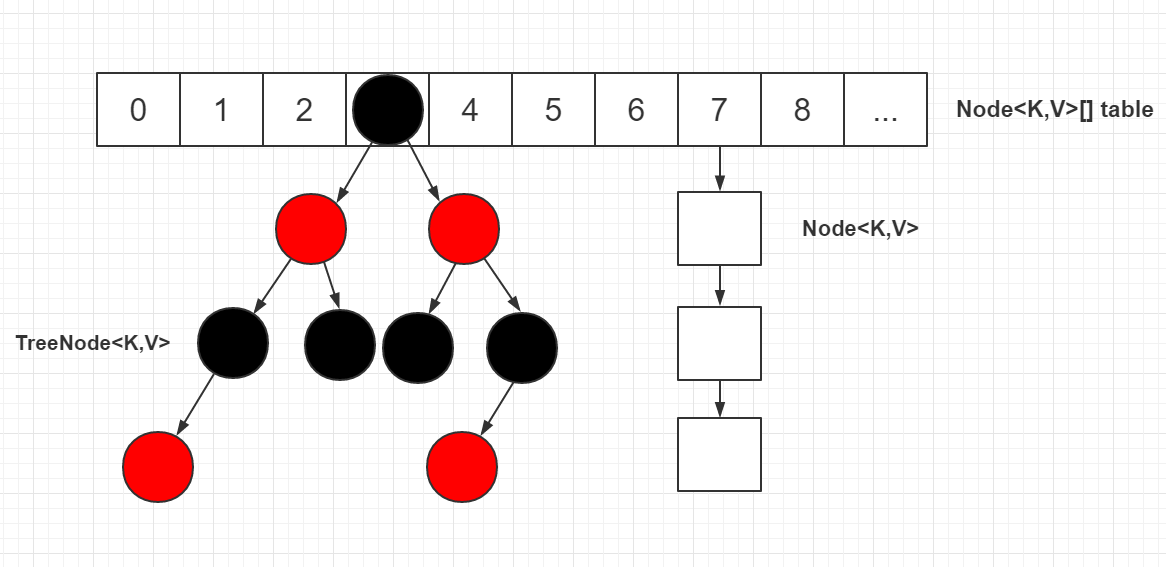# 几个点：

1. 头节点指的是table表上索引位置的节点，也就是链表的头节点。
2. 根结点（root节点）指的是红黑树最上面的那个节点，也就是没有父节点的节点。
3. 红黑树的根结点一定是索引位置的头结点（也就是链表的头结点），通过moveRootToFront方法来维持。
4. 转为红黑树节点后，链表的结构还存在，通过next属性维持，红黑树节点在进行操作时都会维护链表的结构，并不是转为红黑树节点，链表结构就不存在了。
5. 在红黑树上，叶子节点也可能有next节点，因为红黑树的结构跟链表的结构是互不影响的，不会因为是叶子节点就说该节点已经没有next节点。
6. 源码中一些变量定义：如果定义了一个节点p，则pl为p的左节点，pr为p的右节点，pp为p的父节点，ph为p的hash值，pk为p的key值，kc为key的类等等。源码中很喜欢在if/for等语句中进行赋值并判断，请注意。
7. 链表中移除一个节点只需如下图操作，其他操作同理。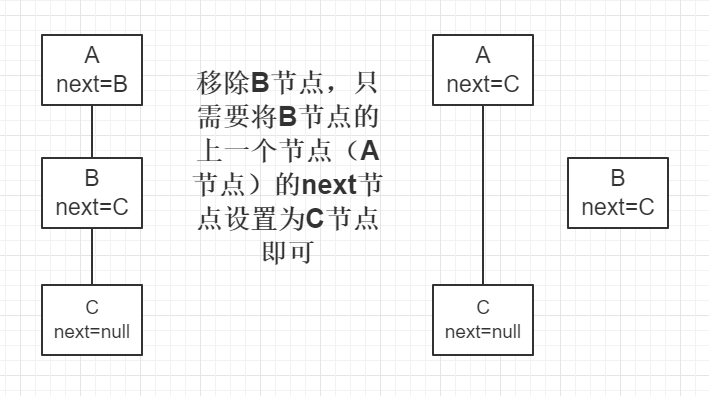8. 红黑树在维护链表结构时，移除一个节点只需如下图操作（红黑树中增加了一个prev属性），其他操作同理。注：此处只是红黑树维护链表结构的操作，红黑树还需要单独进行红黑树的移除或者其他操作。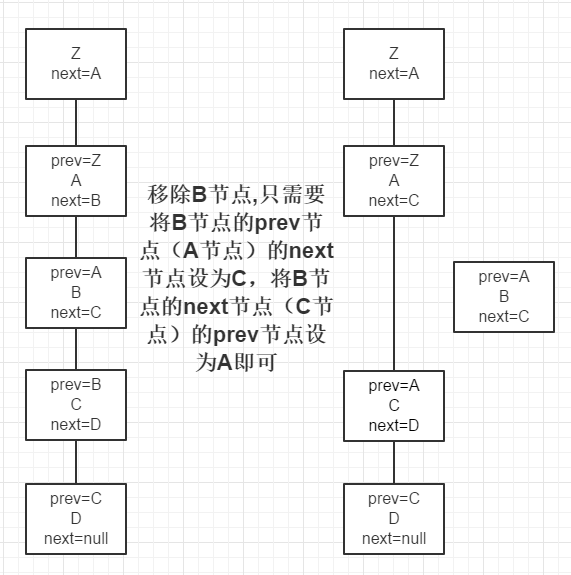9. 源码中进行红黑树的查找时，会反复用到以下两条规则：1）如果目标节点的hash值小于p节点的hash值，则向p节点的左边遍历；否则向p节点的右边遍历。2）如果目标节点的key值小于p节点的key值，则向p节点的左边遍历；否则向p节点的右边遍历。这两条规则是利用了红黑树的特性（左节点<根结点<右节点）。
10. 源码中进行红黑树的查找时，会用dir（direction）来表示向左还是向右查找，dir存储的值是目标节点的hash/key与p节点的hash/key的比较结果。

# 基本属性

``````/**
* The default initial capacity - MUST be a power of two.
*/
static final int DEFAULT_INITIAL_CAPACITY = 1 << 4; // 默认容量16

/**
* The maximum capacity, used if a higher value is implicitly specified
* by either of the constructors with arguments.
* MUST be a power of two <= 1<<30.
*/
static final int MAXIMUM_CAPACITY = 1 << 30;    // 最大容量

/**
* The load factor used when none specified in constructor.
*/
static final float DEFAULT_LOAD_FACTOR = 0.75f; // 默认负载因子0.75

/**
* The bin count threshold for using a tree rather than list for a
* bin.  Bins are converted to trees when adding an element to a
* bin with at least this many nodes. The value must be greater
* than 2 and should be at least 8 to mesh with assumptions in
* tree removal about conversion back to plain bins upon
* shrinkage.
*/
static final int TREEIFY_THRESHOLD = 8; // 链表节点转换红黑树节点的阈值, 9个节点转

/**
* The bin count threshold for untreeifying a (split) bin during a
* resize operation. Should be less than TREEIFY_THRESHOLD, and at
* most 6 to mesh with shrinkage detection under removal.
*/
static final int UNTREEIFY_THRESHOLD = 6;   // 红黑树节点转换链表节点的阈值, 6个节点转

/**
* The smallest table capacity for which bins may be treeified.
* (Otherwise the table is resized if too many nodes in a bin.)
* Should be at least 4 * TREEIFY_THRESHOLD to avoid conflicts
* between resizing and treeification thresholds.
*/
static final int MIN_TREEIFY_CAPACITY = 64; // 转红黑树时, table的最小长度

/**
* Basic hash bin node, used for most entries.  (See below for
* TreeNode subclass, and in LinkedHashMap for its Entry subclass.)
*/
static class Node<K,V> implements Map.Entry<K,V> {  // 基本hash节点, 继承自Entry
final int hash;
final K key;
V value;
Node<K,V> next;

Node(int hash, K key, V value, Node<K,V> next) {
this.hash = hash;
this.key = key;
this.value = value;
this.next = next;
}

public final K getKey()        { return key; }
public final V getValue()      { return value; }
public final String toString() { return key + "=" + value; }

public final int hashCode() {
return Objects.hashCode(key) ^ Objects.hashCode(value);
}

public final V setValue(V newValue) {
V oldValue = value;
value = newValue;
return oldValue;
}

public final boolean equals(Object o) {
if (o == this)
return true;
if (o instanceof Map.Entry) {
Map.Entry<?,?> e = (Map.Entry<?,?>)o;
if (Objects.equals(key, e.getKey()) &&
Objects.equals(value, e.getValue()))
return true;
}
return false;
}
}

/**
* Entry for Tree bins. Extends LinkedHashMap.Entry (which in turn
* extends Node) so can be used as extension of either regular or
*/
static final class TreeNode<K,V> extends LinkedHashMap.Entry<K,V> {// 红黑树节点
TreeNode<K,V> parent;  // red-black tree links
TreeNode<K,V> left;
TreeNode<K,V> right;
TreeNode<K,V> prev;    // needed to unlink next upon deletion
boolean red;
TreeNode(int hash, K key, V val, Node<K,V> next) {
super(hash, key, val, next);
}
// ...
}``````

# 定位哈希桶数组索引位置

``````// 代码1
static final int hash(Object key) { // 计算key的hash值
int h;
// 1.先拿到key的hashCode值; 2.将hashCode的高16位参与运算
return (key == null) ? 0 : (h = key.hashCode()) ^ (h >>> 16);
}
// 代码2
int n = tab.length;
// 将(tab.length - 1) 与 hash值进行&运算
int index = (n - 1) & hash;``````

1. 拿到key的hashCode值
2. 将hashCode的高位参与运算，重新计算hash值
3. 将计算出来的hash值与(table.length – 1)进行&运算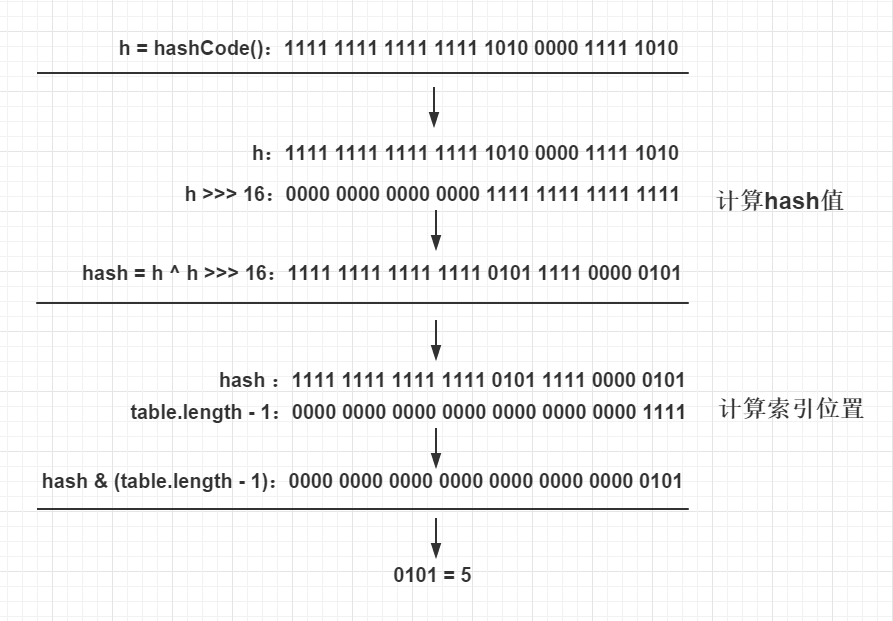# get方法

``````public V get(Object key) {
Node<K,V> e;
return (e = getNode(hash(key), key)) == null ? null : e.value;
}

final Node<K,V> getNode(int hash, Object key) {
Node<K,V>[] tab; Node<K,V> first, e; int n; K k;
// table不为空 && table长度大于0 && table索引位置(根据hash值计算出)不为空
if ((tab = table) != null && (n = tab.length) > 0 &&
(first = tab[(n - 1) & hash]) != null) {
if (first.hash == hash && // always check first node
((k = first.key) == key || (key != null && key.equals(k))))
return first;	// first的key等于传入的key则返回first对象
if ((e = first.next) != null) { // 向下遍历
if (first instanceof TreeNode)  // 判断是否为TreeNode
// 如果是红黑树节点，则调用红黑树的查找目标节点方法getTreeNode
return ((TreeNode<K,V>)first).getTreeNode(hash, key);
// 走到这代表节点为链表节点
do { // 向下遍历链表, 直至找到节点的key和传入的key相等时,返回该节点
if (e.hash == hash &&
((k = e.key) == key || (key != null && key.equals(k))))
return e;
} while ((e = e.next) != null);
}
}
return null;    // 找不到符合的返回空
}``````
1. 先对table进行校验，校验是否为空，length是否大于0
2. 使用table.length – 1和hash值进行位与运算，得出在table上的索引位置，将该索引位置的节点赋值给first节点，校验该索引位置是否为空
3. 检查first节点的hash值和key是否和入参的一样，如果一样则first即为目标节点，直接返回first节点
4. 如果first的next节点不为空则继续遍历
5. 如果first节点为TreeNode，则调用getTreeNode方法（见下文代码块1）查找目标节点
6. 如果first节点不为TreeNode，则调用普通的遍历链表方法查找目标节点
7. 如果查找不到目标节点则返回空

## 代码块1：getTreeNode方法

``````final TreeNode<K,V> getTreeNode(int h, Object k) {
// 使用根结点调用find方法
return ((parent != null) ? root() : this).find(h, k, null);
}``````
1. 找到调用此方法的节点的树的根节点
2. 使用该树的根节点调用find方法（见下文代码块2）

## 代码块2：find方法

``````/**
* 从调用此方法的结点开始查找, 通过hash值和key找到对应的节点
* 此处是红黑树的遍历, 红黑树是特殊的自平衡二叉查找树
* 平衡二叉查找树的特点：左节点<根节点<右节点
*/
final TreeNode<K,V> find(int h, Object k, Class<?> kc) {
TreeNode<K,V> p = this; // this为调用此方法的节点
do {
int ph, dir; K pk;
TreeNode<K,V> pl = p.left, pr = p.right, q;
if ((ph = p.hash) > h)  // 传入的hash值小于p节点的hash值, 则往p节点的左边遍历
p = pl; // p赋值为p节点的左节点
else if (ph < h)    // 传入的hash值大于p节点的hash值, 则往p节点的右边遍历
p = pr; // p赋值为p节点的右节点
// 传入的hash值和key值等于p节点的hash值和key值,则p节点为目标节点,返回p节点
else if ((pk = p.key) == k || (k != null && k.equals(pk)))
return p;
else if (pl == null)    // p节点的左节点为空则将向右遍历
p = pr;
else if (pr == null)    // p节点的右节点为空则向左遍历
p = pl;
else if ((kc != null ||
// 如果传入的key(k)所属的类实现了Comparable接口,则将传入的key跟p节点的key比较
(kc = comparableClassFor(k)) != null) && // 此行不为空代表k实现了Comparable
(dir = compareComparables(kc, k, pk)) != 0)//k<pk则dir<0, k>pk则dir>0
p = (dir < 0) ? pl : pr;    // k < pk则向左遍历(p赋值为p的左节点), 否则向右遍历
// 代码走到此处, 代表key所属类没有实现Comparable, 直接指定向p的右边遍历
else if ((q = pr.find(h, k, kc)) != null)
return q;
else// 代码走到此处代表上一个向右遍历（pr.find(h, k, kc)）为空, 因此直接向左遍历
p = pl;
} while (p != null);
return null;
}``````
1. 将p节点赋值为调用此方法的节点
2. 如果传入的hash值小于p节点的hash值，则往p节点的左边遍历
3. 如果传入的hash值大于p节点的hash值，则往p节点的右边遍历
4. 如果传入的hash值等于p节点的hash值，并且传入的key值跟p节点的key值相等, 则该p节点即为目标节点，返回p节点
5. 如果p的左节点为空则向右遍历，反之如果p的右节点为空则向左遍历
6. 如果传入的key（即代码中的参数变量k）所属的类实现了Comparable接口（kc不为空，comparableClassFor方法见下文代码块3），则将传入的key跟p节点的key进行比较（kc实现了Comparable接口，因此通过kc的比较方法进行比较），并将比较结果赋值给dir，如果dir<0则代表k<pk，则向p节点的左边遍历（pl）；否则，向p节点的右边遍历（pr）。
7. 代码走到此处，代表key所属类没有实现Comparable，因此直接指定向p的右边遍历，如果能找到目标节点则返回
8. 代码走到此处代表与第7点向右遍历没有找到目标节点，因此直接向左边遍历
9. 以上都找不到目标节点则返回空

## 代码块3：comparableClassFor方法

``````/**
* Returns x's Class if it is of the form "class C implements
* Comparable<C>", else null.
*/
static Class<?> comparableClassFor(Object x) {
if (x instanceof Comparable) {
Class<?> c; Type[] ts, as; Type t; ParameterizedType p;
if ((c = x.getClass()) == String.class) // bypass checks
return c;
if ((ts = c.getGenericInterfaces()) != null) {
for (int i = 0; i < ts.length; ++i) {
if (((t = ts[i]) instanceof ParameterizedType) &&
((p = (ParameterizedType)t).getRawType() ==
Comparable.class) &&
(as = p.getActualTypeArguments()) != null &&
as.length == 1 && as == c) // type arg is c
return c;
}
}
}
return null;
}``````

# put方法

``````public V put(K key, V value) {
return putVal(hash(key), key, value, false, true);
}

final V putVal(int hash, K key, V value, boolean onlyIfAbsent,
boolean evict) {
Node<K,V>[] tab; Node<K,V> p; int n, i;
// table是否为空或者length等于0, 如果是则调用resize方法进行初始化
if ((tab = table) == null || (n = tab.length) == 0)
n = (tab = resize()).length;
// 通过hash值计算索引位置, 如果table表该索引位置节点为空则新增一个
if ((p = tab[i = (n - 1) & hash]) == null)// 将索引位置的头节点赋值给p
tab[i] = newNode(hash, key, value, null);
else {  // table表该索引位置不为空
Node<K,V> e; K k;
if (p.hash == hash && // 判断p节点的hash值和key值是否跟传入的hash值和key值相等
((k = p.key) == key || (key != null && key.equals(k))))
e = p;  // 如果相等, 则p节点即为要查找的目标节点，赋值给e
// 判断p节点是否为TreeNode, 如果是则调用红黑树的putTreeVal方法查找目标节点
else if (p instanceof TreeNode)
e = ((TreeNode<K,V>)p).putTreeVal(this, tab, hash, key, value);
else {	// 走到这代表p节点为普通链表节点
for (int binCount = 0; ; ++binCount) {  // 遍历此链表, binCount用于统计节点数
if ((e = p.next) == null) { // p.next为空代表不存在目标节点则新增一个节点插入链表尾部
p.next = newNode(hash, key, value, null);
// 计算节点是否超过8个, 减一是因为循环是从p节点的下一个节点开始的
if (binCount >= TREEIFY_THRESHOLD - 1)
treeifyBin(tab, hash);// 如果超过8个，调用treeifyBin方法将该链表转换为红黑树
break;
}
if (e.hash == hash && // e节点的hash值和key值都与传入的相等, 则e即为目标节点,跳出循环
((k = e.key) == key || (key != null && key.equals(k))))
break;
p = e;  // 将p指向下一个节点
}
}
// e不为空则代表根据传入的hash值和key值查找到了节点,将该节点的value覆盖,返回oldValue
if (e != null) {
V oldValue = e.value;
if (!onlyIfAbsent || oldValue == null)
e.value = value;
return oldValue;
}
}
++modCount;
if (++size > threshold) // 插入节点后超过阈值则进行扩容
resize();
return null;
}``````
1. 校验table是否为空或者length等于0，如果是则调用resize方法（见下文resize方法）进行初始化
2. 通过hash值计算索引位置，将该索引位置的头节点赋值给p节点，如果该索引位置节点为空则使用传入的参数新增一个节点并放在该索引位置
3. 判断p节点的key和hash值是否跟传入的相等，如果相等, 则p节点即为要查找的目标节点，将p节点赋值给e节点
4. 如果p节点不是目标节点，则判断p节点是否为TreeNode，如果是则调用红黑树的putTreeVal方法（见下文代码块4）查找目标节点
5. 走到这代表p节点为普通链表节点，则调用普通的链表方法进行查找，并定义变量binCount来统计该链表的节点数
6. 如果p的next节点为空时，则代表找不到目标节点，则新增一个节点并插入链表尾部，并校验节点数是否超过8个，如果超过则调用treeifyBin方法（见下文代码块6）将链表节点转为红黑树节点
7. 如果遍历的e节点存在hash值和key值都与传入的相同，则e节点即为目标节点，跳出循环
8. 如果e节点不为空，则代表目标节点存在，使用传入的value覆盖该节点的value，并返回oldValue
9. 如果插入节点后节点数超过阈值，则调用resize方法（见下文resize方法）进行扩容

## 代码块4：putTreeVal方法

``````/**
* Tree version of putVal.
* 红黑树插入会同时维护原来的链表属性, 即原来的next属性
*/
final TreeNode<K,V> putTreeVal(HashMap<K,V> map, Node<K,V>[] tab,
int h, K k, V v) {
Class<?> kc = null;
boolean searched = false;
// 查找根节点, 索引位置的头节点并不一定为红黑树的根结点
TreeNode<K,V> root = (parent != null) ? root() : this;
for (TreeNode<K,V> p = root;;) {    // 将根节点赋值给p, 开始遍历
int dir, ph; K pk;
if ((ph = p.hash) > h)  // 如果传入的hash值小于p节点的hash值
dir = -1;	// 则将dir赋值为-1, 代表向p的左边查找树
else if (ph < h)    // 如果传入的hash值大于p节点的hash值,
dir = 1;	// 则将dir赋值为1, 代表向p的右边查找树
// 如果传入的hash值和key值等于p节点的hash值和key值, 则p节点即为目标节点, 返回p节点
else if ((pk = p.key) == k || (k != null && k.equals(pk)))
return p;
// 如果k所属的类没有实现Comparable接口 或者 k和p节点的key相等
else if ((kc == null &&
(kc = comparableClassFor(k)) == null) ||
(dir = compareComparables(kc, k, pk)) == 0) {
if (!searched) {    // 第一次符合条件, 该方法只有第一次才执行
TreeNode<K,V> q, ch;
searched = true;
// 从p节点的左节点和右节点分别调用find方法进行查找, 如果查找到目标节点则返回
if (((ch = p.left) != null &&
(q = ch.find(h, k, kc)) != null) ||
((ch = p.right) != null &&
(q = ch.find(h, k, kc)) != null))
return q;
}
// 否则使用定义的一套规则来比较k和p节点的key的大小, 用来决定向左还是向右查找
dir = tieBreakOrder(k, pk); // dir<0则代表k<pk，则向p左边查找；反之亦然
}

TreeNode<K,V> xp = p;   // xp赋值为x的父节点,中间变量,用于下面给x的父节点赋值
// dir<=0则向p左边查找,否则向p右边查找,如果为null,则代表该位置即为x的目标位置
if ((p = (dir <= 0) ? p.left : p.right) == null) {
// 走进来代表已经找到x的位置，只需将x放到该位置即可
Node<K,V> xpn = xp.next;    // xp的next节点
// 创建新的节点, 其中x的next节点为xpn, 即将x节点插入xp与xpn之间
TreeNode<K,V> x = map.newTreeNode(h, k, v, xpn);
if (dir <= 0)   // 如果时dir <= 0, 则代表x节点为xp的左节点
xp.left = x;
else        // 如果时dir> 0, 则代表x节点为xp的右节点
xp.right = x;
xp.next = x;    // 将xp的next节点设置为x
x.parent = x.prev = xp; // 将x的parent和prev节点设置为xp
// 如果xpn不为空,则将xpn的prev节点设置为x节点,与上文的x节点的next节点对应
if (xpn != null)
((TreeNode<K,V>)xpn).prev = x;
moveRootToFront(tab, balanceInsertion(root, x)); // 进行红黑树的插入平衡调整
return null;
}
}
}``````
1. 查找当前红黑树的根结点，将根结点赋值给p节点，开始进行查找
2. 如果传入的hash值小于p节点的hash值，将dir赋值为-1，代表向p的左边查找树
3. 如果传入的hash值大于p节点的hash值， 将dir赋值为1，代表向p的右边查找树
4. 如果传入的hash值等于p节点的hash值，并且传入的key值跟p节点的key值相等, 则该p节点即为目标节点，返回p节点
5. 如果k所属的类没有实现Comparable接口，或者k和p节点的key使用compareTo方法比较相等：第一次会从p节点的左节点和右节点分别调用find方法（见上文代码块2）进行查找，如果查找到目标节点则返回；如果不是第一次或者调用find方法没有找到目标节点，则调用tieBreakOrder方法（见下文代码块5）比较k和p节点的key值的大小，以决定向树的左节点还是右节点查找。
6. 如果dir <= 0则向左节点查找（p赋值为p.left，并进行下一次循环），否则向右节点查找，如果已经无法继续查找（p赋值后为null），则代表该位置即为x的目标位置，另外变量xp用来记录查找的最后一个节点，即下文新增的x节点的父节点。
7. 以传入的hash、key、value参数和xp节点的next节点为参数，构建x节点（注意：xp节点在此处可能是叶子节点、没有左节点的节点、没有右节点的节点三种情况，即使它是叶子节点，它也可能有next节点，红黑树的结构跟链表的结构是互不影响的，不会因为某个节点是叶子节点就说它没有next节点，红黑树在进行操作时会同时维护红黑树结构和链表结构，next属性就是用来维护链表结构的），根据dir的值决定x决定放在xp节点的左节点还是右节点，将xp的next节点设为x，将x的parent和prev节点设为xp，如果原xp的next节点（xpn）不为空, 则将该节点的prev节点设置为x节点, 与上面的将x节点的next节点设置为xpn对应。
8. 进行红黑树的插入平衡调整，见文末的解释2。

## 代码块5：tieBreakOrder方法

``````// 用于不可比较或者hashCode相同时进行比较的方法, 只是一个一致的插入规则，用来维护重定位的等价性。
static int tieBreakOrder(Object a, Object b) {
int d;
if (a == null || b == null ||
(d = a.getClass().getName().
compareTo(b.getClass().getName())) == 0)
d = (System.identityHashCode(a) <= System.identityHashCode(b) ?
-1 : 1);
return d;
}``````

## 代码块6：treeifyBin方法

``````final void treeifyBin(Node<K,V>[] tab, int hash) {
int n, index; Node<K,V> e;
// table为空或者table的长度小于64, 进行扩容
if (tab == null || (n = tab.length) < MIN_TREEIFY_CAPACITY)
resize();
// 根据hash值计算索引值, 遍历该索引位置的链表
else if ((e = tab[index = (n - 1) & hash]) != null) {
TreeNode<K,V> hd = null, tl = null;
do {
TreeNode<K,V> p = replacementTreeNode(e, null); // 链表节点转红黑树节点
if (tl == null)	// tl为空代表为第一次循环
hd = p; // 头结点
else {
p.prev = tl;    // 当前节点的prev属性设为上一个节点
tl.next = p;    // 上一个节点的next属性设置为当前节点
}
tl = p; // tl赋值为p, 在下一次循环中作为上一个节点
} while ((e = e.next) != null);	// e指向下一个节点
// 将table该索引位置赋值为新转的TreeNode的头节点
if ((tab[index] = hd) != null)
hd.treeify(tab);    // 以头结点为根结点, 构建红黑树
}
}``````
1. 校验table是否为空，如果长度小于64，则调用resize方法（见下文resize方法）进行扩容。
2. 根据hash值计算索引值，将该索引位置的节点赋值给e节点，从e节点开始遍历该索引位置的链表。
3. 调用replacementTreeNode方法（该方法就一行代码，直接返回一个新建的TreeNode）将链表节点转为红黑树节点，将头结点赋值给hd节点，每次遍历结束将p节点赋值给tl，用于在下一次循环中作为上一个节点进行一些链表的关联操作（p.prev = tl 和 tl.next = p）。
4. 将table该索引位置赋值为新转的TreeNode的头节点hd，如果该节点不为空，则以hd为根结点，调用treeify方法（见下文代码块7）构建红黑树。

## 代码块7：treeify方法

``````final void treeify(Node<K,V>[] tab) {   // 构建红黑树
TreeNode<K,V> root = null;
for (TreeNode<K,V> x = this, next; x != null; x = next) {// this即为调用此方法的TreeNode
next = (TreeNode<K,V>)x.next;   // next赋值为x的下个节点
x.left = x.right = null;    // 将x的左右节点设置为空
if (root == null) { // 如果还没有根结点, 则将x设置为根结点
x.parent = null;    // 根结点没有父节点
x.red = false;  // 根结点必须为黑色
root = x;   // 将x设置为根结点
}
else {
K k = x.key;	// k赋值为x的key
int h = x.hash;	// h赋值为x的hash值
Class<?> kc = null;
// 如果当前节点x不是根结点, 则从根节点开始查找属于该节点的位置
for (TreeNode<K,V> p = root;;) {
int dir, ph;
K pk = p.key;
if ((ph = p.hash) > h)  // 如果x节点的hash值小于p节点的hash值
dir = -1;   // 则将dir赋值为-1, 代表向p的左边查找
else if (ph < h)    // 与上面相反, 如果x节点的hash值大于p节点的hash值
dir = 1;    // 则将dir赋值为1, 代表向p的右边查找
// 走到这代表x的hash值和p的hash值相等，则比较key值
else if ((kc == null && // 如果k没有实现Comparable接口 或者 x节点的key和p节点的key相等
(kc = comparableClassFor(k)) == null) ||
(dir = compareComparables(kc, k, pk)) == 0)
// 使用定义的一套规则来比较x节点和p节点的大小，用来决定向左还是向右查找
dir = tieBreakOrder(k, pk);

TreeNode<K,V> xp = p;   // xp赋值为x的父节点,中间变量用于下面给x的父节点赋值
// dir<=0则向p左边查找,否则向p右边查找,如果为null,则代表该位置即为x的目标位置
if ((p = (dir <= 0) ? p.left : p.right) == null) {
x.parent = xp;  // x的父节点即为最后一次遍历的p节点
if (dir <= 0)   // 如果时dir <= 0, 则代表x节点为父节点的左节点
xp.left = x;
else    // 如果时dir > 0, 则代表x节点为父节点的右节点
xp.right = x;
// 进行红黑树的插入平衡(通过左旋、右旋和改变节点颜色来保证当前树符合红黑树的要求)
root = balanceInsertion(root, x);
break;
}
}
}
}
moveRootToFront(tab, root); // 如果root节点不在table索引位置的头结点, 则将其调整为头结点
}``````
1. 从调用此方法的节点作为起点，开始进行遍历，并将此节点设为root节点，标记为黑色（x.red = false）。
2. 如果当前节点不是根结点，则从根节点开始查找属于该节点的位置（该段代码跟之前的代码块2和代码块4的查找代码类似）。
3. 如果x节点(将要插入红黑树的节点)的hash值小于p节点(当前遍历到的红黑树节点)的hash值，则向p节点的左边查找。
4. 与3相反，如果x节点的hash值大于p节点的hash值，则向p节点的右边查找。
5. 如果x的key没有实现Comparable接口，或者x节点的key和p节点的key相等，使用tieBreakOrder方法（见上文代码块5）来比较x节点和p节点的大小，以决定向左还是向右查找（dir <= 0向左，否则向右）。
6. 如果dir <= 0则向左节点查找（p赋值为p.left，并进行下一次循环），否则向右节点查找，如果已经无法继续查找（p赋值后为null），则代表该位置即为x的目标位置，另外变量xp用来记录最后一个节点，即为下文新增的x节点的父节点。
7. 将x的父节点设置为xp，根据dir的值决定x决定放在xp节点的左节点还是右节点，最后进行红黑树的插入平衡调整。
8. 调用moveRootToFront方法（见下文代码块8）将root节点调整到索引位置的头结点。

## 代码块8：moveRootToFront方法

``````/**
* 如果当前索引位置的头节点不是root节点, 则将root的上一个节点和下一个节点进行关联,
* 将root放到头节点的位置, 原头节点放在root的next节点上
*/
static <K,V> void moveRootToFront(Node<K,V>[] tab, TreeNode<K,V> root) {
int n;
if (root != null && tab != null && (n = tab.length) > 0) {
int index = (n - 1) & root.hash;
TreeNode<K,V> first = (TreeNode<K,V>)tab[index];
if (root != first) {    // 如果root节点不是该索引位置的头节点
Node<K,V> rn;
tab[index] = root;  // 将该索引位置的头节点赋值为root节点
TreeNode<K,V> rp = root.prev;   // root节点的上一个节点
// 如果root节点的下一个节点不为空,
// 则将root节点的下一个节点的prev属性设置为root节点的上一个节点
if ((rn = root.next) != null)
((TreeNode<K,V>)rn).prev = rp;
// 如果root节点的上一个节点不为空,
// 则将root节点的上一个节点的next属性设置为root节点的下一个节点
if (rp != null)
rp.next = rn;
if (first != null)  // 如果原头节点不为空, 则将原头节点的prev属性设置为root节点
first.prev = root;
root.next = first;  // 将root节点的next属性设置为原头节点
root.prev = null;
}
assert checkInvariants(root);   // 检查树是否正常
}
}``````
1. 校验root是否为空、table是否为空、table的length是否大于0。
2. 根据root节点的hash值计算出索引位置，判断该索引位置的头节点是否为root节点，如果不是则进行以下操作将该索引位置的头结点替换为root节点。
3. 将该索引位置的头结点赋值为root节点，如果root节点的next节点不为空，则将root节点的next节点的prev属性设置为root节点的prev节点。
4. 如果root节点的prev节点不为空，则将root节点的prev节点的next属性设置为root节点的next节点（3和4两个操作是一个完整的链表移除某个节点过程）。
5. 如果原头节点不为空，则将原头节点的prev属性设置为root节点
6. 将root节点的next属性设置为原头节点（5和6两个操作将first节点接到root节点后面）
7. root此时已经被放到该位置的头结点位置，因此将prev属性设为空。
8. 调用checkInvariants方法（见下文代码块9）检查树是否正常。

## 代码块9：checkInvariants方法

``````/**
* Recursive invariant check
*/
static <K,V> boolean checkInvariants(TreeNode<K,V> t) { // 一些基本的校验
TreeNode<K,V> tp = t.parent, tl = t.left, tr = t.right,
tb = t.prev, tn = (TreeNode<K,V>)t.next;
if (tb != null && tb.next != t)
return false;
if (tn != null && tn.prev != t)
return false;
if (tp != null && t != tp.left && t != tp.right)
return false;
if (tl != null && (tl.parent != t || tl.hash > t.hash))
return false;
if (tr != null && (tr.parent != t || tr.hash < t.hash))
return false;
if (t.red && tl != null && tl.red && tr != null && tr.red)  // 如果当前节点为红色, 则该节点的左右节点都不能为红色
return false;
if (tl != null && !checkInvariants(tl))
return false;
if (tr != null && !checkInvariants(tr))
return false;
return true;
}``````

# resize方法

``````final Node<K,V>[] resize() {
Node<K,V>[] oldTab = table;
int oldCap = (oldTab == null) ? 0 : oldTab.length;
int oldThr = threshold;
int newCap, newThr = 0;
if (oldCap > 0) {   // 老table不为空
if (oldCap >= MAXIMUM_CAPACITY) {      // 老table的容量超过最大容量值
threshold = Integer.MAX_VALUE;  // 设置阈值为Integer.MAX_VALUE
return oldTab;
}
// 将新容量赋值为老容量*2，如果新容量<最大容量并且老容量>=16, 则将新阈值设置为原来的两倍
else if ((newCap = oldCap << 1) < MAXIMUM_CAPACITY &&
oldCap >= DEFAULT_INITIAL_CAPACITY)
newThr = oldThr << 1; // double threshold
}
else if (oldThr > 0) // 老表的容量为0, 老表的阈值大于0, 是因为初始容量被放入阈值
newCap = oldThr;	// 则将新表的容量设置为老表的阈值
else {	// 老表的容量为0, 老表的阈值为0, 则为空表，设置默认容量和阈值
newCap = DEFAULT_INITIAL_CAPACITY;
}
if (newThr == 0) {  // 如果新阈值为空, 则通过新的容量*负载因子获得新阈值
float ft = (float)newCap * loadFactor;
newThr = (newCap < MAXIMUM_CAPACITY && ft < (float)MAXIMUM_CAPACITY ?
(int)ft : Integer.MAX_VALUE);
}
threshold = newThr; // 将当前阈值赋值为刚计算出来的新的阈值
@SuppressWarnings({"rawtypes","unchecked"})
// 定义新表,容量为刚计算出来的新容量
Node<K,V>[] newTab = (Node<K,V>[])new Node[newCap];
table = newTab; // 将当前的表赋值为新定义的表
if (oldTab != null) {   // 如果老表不为空, 则需遍历将节点赋值给新表
for (int j = 0; j < oldCap; ++j) {
Node<K,V> e;
if ((e = oldTab[j]) != null) {  // 将索引值为j的老表头节点赋值给e
oldTab[j] = null; // 将老表的节点设置为空, 以便垃圾收集器回收空间
// 如果e.next为空, 则代表老表的该位置只有1个节点,
// 通过hash值计算新表的索引位置, 直接将该节点放在该位置
if (e.next == null)
newTab[e.hash & (newCap - 1)] = e;
else if (e instanceof TreeNode)
// 调用treeNode的hash分布(跟下面最后一个else的内容几乎相同)
((TreeNode<K,V>)e).split(this, newTab, j, oldCap);
else { // preserve order
Node<K,V> loHead = null, loTail = null; // 存储跟原索引位置相同的节点
Node<K,V> hiHead = null, hiTail = null; // 存储索引位置为:原索引+oldCap的节点
Node<K,V> next;
do {
next = e.next;
//如果e的hash值与老表的容量进行与运算为0,则扩容后的索引位置跟老表的索引位置一样
if ((e.hash & oldCap) == 0) {
if (loTail == null) // 如果loTail为空, 代表该节点为第一个节点
else
loTail.next = e;    // 否则将节点添加在loTail后面
loTail = e; // 并将loTail赋值为新增的节点
}
//如果e的hash值与老表的容量进行与运算为1,则扩容后的索引位置为:老表的索引位置＋oldCap
else {
if (hiTail == null) // 如果hiTail为空, 代表该节点为第一个节点
else
hiTail.next = e;    // 否则将节点添加在hiTail后面
hiTail = e; // 并将hiTail赋值为新增的节点
}
} while ((e = next) != null);
if (loTail != null) {
loTail.next = null; // 最后一个节点的next设为空
}
if (hiTail != null) {
hiTail.next = null; // 最后一个节点的next设为空
newTab[j + oldCap] = hiHead; // 将索引位置为原索引+oldCap的节点设置为对应的头结点
}
}
}
}
}
return newTab;
}``````
1. 如果老表的容量大于0，判断老表的容量是否超过最大容量值：如果超过则将阈值设置为Integer.MAX_VALUE，并直接返回老表（此时oldCap * 2比Integer.MAX_VALUE大，因此无法进行重新分布，只是单纯的将阈值扩容到最大）；将新表的容量赋值为老表的容量*2，如果新容量小于最大容量并且老容量不小于16，则直接将新的阈值设置为原来的两倍。
3. 如果老表的容量为0，老表的阈值为0，这种情况是没有传容量的new方法创建的空表，将阈值和容量设置为默认值。
4. 如果新表的阈值为空，则通过新的容量 * 负载因子获得阈值（这种情况是初始化的时候传了初始容量，跟第2点相同情况，或者初始容量设置的太小导致老表的容量没有超过16导致的）。
5. 将当前阈值设置为刚计算出来的新的阈值，定义新表，容量为刚计算出来的新容量，将当前的表设置为新定义的表。
6. 如果老表不为空，则需遍历所有节点，将节点赋值给新表。
7. 将老表上索引为j的头结点赋值给e节点，并将老表上索引为j的节点设置为空。
8. 如果e的next节点为空，则代表老表的该位置只有1个节点，通过hash值计算新表的索引位置，直接将该节点放在新表的该位置上。
9. 如果e的next节点不为空，并且e为TreeNode，则调用split方法（见下文代码块10）进行hash分布。
10. 如果e的next节点不为空，并且e为普通的链表节点，则进行普通的hash分布。
13. 老表节点重新hash分布在新表结束后，如果loTail不为空（说明老表的数据有分布到新表上原索引位置的节点），则将最后一个节点的next设为空，并将新表上原索引位置的节点设置为对应的头结点；如果hiTail不为空（说明老表的数据有分布到新表上原索引+oldCap位置的节点），则将最后一个节点的next设为空，并将新表上索引位置为原索引+oldCap的节点设置为对应的头结点。
14. 返回新表。

## 代码块10：split方法

``````final void split(HashMap<K,V> map, Node<K,V>[] tab, int index, int bit) {
TreeNode<K,V> b = this;	// 拿到调用此方法的节点
TreeNode<K,V> loHead = null, loTail = null; // 存储跟原索引位置相同的节点
TreeNode<K,V> hiHead = null, hiTail = null; // 存储索引位置为:原索引+oldCap的节点
int lc = 0, hc = 0;
for (TreeNode<K,V> e = b, next; e != null; e = next) {	// 从b节点开始遍历
next = (TreeNode<K,V>)e.next;   // next赋值为e的下个节点
e.next = null;  // 同时将老表的节点设置为空，以便垃圾收集器回收
//如果e的hash值与老表的容量进行与运算为0,则扩容后的索引位置跟老表的索引位置一样
if ((e.hash & bit) == 0) {
if ((e.prev = loTail) == null)  // 如果loTail为空, 代表该节点为第一个节点
else
loTail.next = e;    // 否则将节点添加在loTail后面
loTail = e; // 并将loTail赋值为新增的节点
++lc;   // 统计原索引位置的节点个数
}
//如果e的hash值与老表的容量进行与运算为1,则扩容后的索引位置为:老表的索引位置＋oldCap
else {
if ((e.prev = hiTail) == null)  // 如果hiHead为空, 代表该节点为第一个节点
else
hiTail.next = e;    // 否则将节点添加在hiTail后面
hiTail = e; // 并将hiTail赋值为新增的节点
++hc;   // 统计索引位置为原索引+oldCap的节点个数
}
}

if (loHead != null) {   // 原索引位置的节点不为空
if (lc <= UNTREEIFY_THRESHOLD)  // 节点个数少于6个则将红黑树转为链表结构
else {
// 已经被改变, 需要重新构建新的红黑树
}
}
if (hiHead != null) {   // 索引位置为原索引+oldCap的节点不为空
if (hc <= UNTREEIFY_THRESHOLD)  // 节点个数少于6个则将红黑树转为链表结构
else {
tab[index + bit] = hiHead;  // 将索引位置为原索引+oldCap的节点设置为对应的头结点
// 已经被改变, 需要重新构建新的红黑树
}
}
}``````
1. 以调用此方法的节点开始，遍历整个红黑树节点（此处实际是遍历的链表节点，上文提过，红黑树节点也会同时维护链表结构）。

## 代码块11：untreeify方法

``````// 将红黑树节点转为链表节点, 当节点<=6个时会被触发
final Node<K,V> untreeify(HashMap<K,V> map) {
Node<K,V> hd = null, tl = null; // hd指向头结点, tl指向尾节点
// 从调用该方法的节点, 即链表的头结点开始遍历, 将所有节点全转为链表节点
for (Node<K,V> q = this; q != null; q = q.next) {
// 调用replacementNode方法构建链表节点
Node<K,V> p = map.replacementNode(q, null);
// 如果tl为null, 则代表当前节点为第一个节点, 将hd赋值为该节点
if (tl == null)
hd = p;
else    // 否则, 将尾节点的next属性设置为当前节点p
tl.next = p;
tl = p; // 每次都将tl节点指向当前节点, 即尾节点
}
return hd;  // 返回转换后的链表的头结点
}``````
1. 从调用该方法的节点，即链表的头结点开始遍历, 将所有节点全转为链表节点
2. 调用replacementNode方法构建链表节点
3. 如果tl为null, 则代表当前节点为第一个节点，将hd赋值为该节点，否则, 将尾节点的next属性设置为当前节点p
4. 每次都将tl节点指向当前节点, 即尾节点
5. 返回转换后的链表的头结点

## 例子1：扩容后，节点重hash为什么只可能分布在原索引位置与原索引+oldCap位置？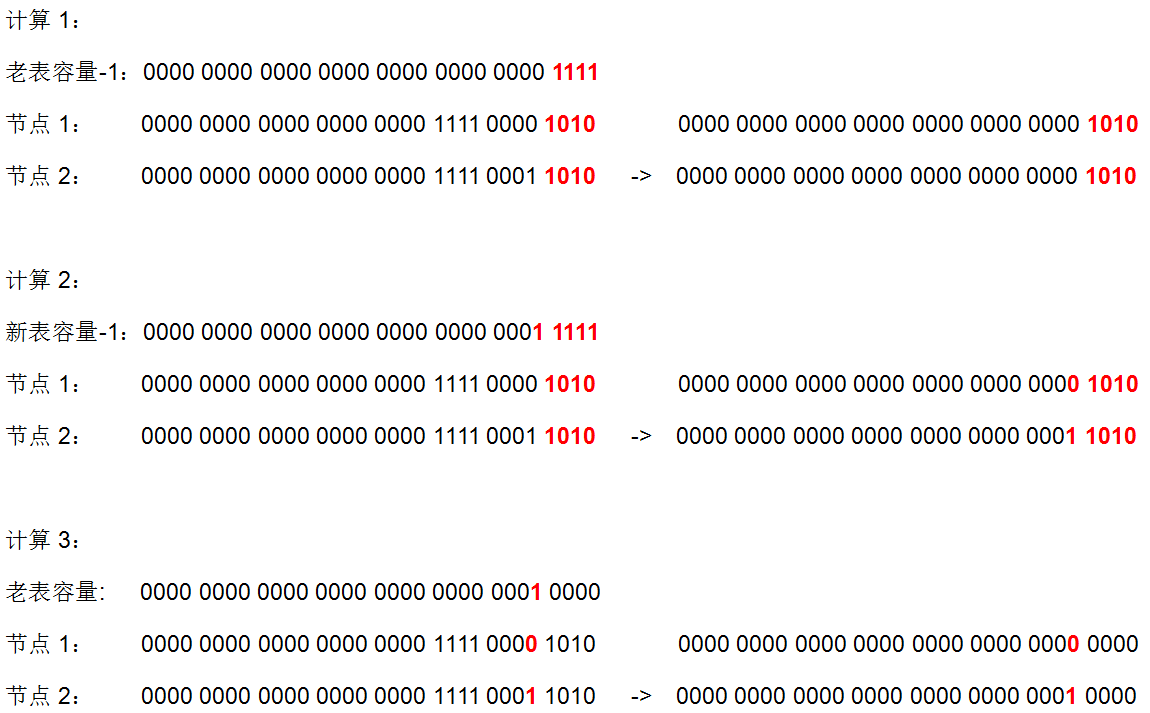# remove方法

``````public V remove(Object key) {
Node<K,V> e;
return (e = removeNode(hash(key), key, null, false, true)) == null ?
null : e.value;
}

final Node<K,V> removeNode(int hash, Object key, Object value,
boolean matchValue, boolean movable) {
Node<K,V>[] tab; Node<K,V> p; int n, index;
// 如果table不为空并且根据hash值计算出来的索引位置不为空, 将该位置的节点赋值给p
if ((tab = table) != null && (n = tab.length) > 0 &&
(p = tab[index = (n - 1) & hash]) != null) {
Node<K,V> node = null, e; K k; V v;
// 如果p的hash值和key都与入参的相同, 则p即为目标节点, 赋值给node
if (p.hash == hash &&
((k = p.key) == key || (key != null && key.equals(k))))
node = p;
else if ((e = p.next) != null) {    // 否则向下遍历节点
if (p instanceof TreeNode)  // 如果p是TreeNode则调用红黑树的方法查找节点
node = ((TreeNode<K,V>)p).getTreeNode(hash, key);
else {
do {    // 遍历链表查找符合条件的节点
// 当节点的hash值和key与传入的相同,则该节点即为目标节点
if (e.hash == hash &&
((k = e.key) == key ||
(key != null && key.equals(k)))) {
node = e;	// 赋值给node, 并跳出循环
break;
}
p = e;  // p节点赋值为本次结束的e
} while ((e = e.next) != null); // 指向像一个节点
}
}
// 如果node不为空(即根据传入key和hash值查找到目标节点)，则进行移除操作
if (node != null && (!matchValue || (v = node.value) == value ||
(value != null && value.equals(v)))) {
if (node instanceof TreeNode)   // 如果是TreeNode则调用红黑树的移除方法
((TreeNode<K,V>)node).removeTreeNode(this, tab, movable);
// 走到这代表节点是普通链表节点
// 如果node是该索引位置的头结点则直接将该索引位置的值赋值为node的next节点
else if (node == p)
tab[index] = node.next;
// 否则将node的上一个节点的next属性设置为node的next节点,
// 即将node节点移除, 将node的上下节点进行关联(链表的移除)
else
p.next = node.next;
++modCount; // 修改次数+1
--size; // table的总节点数-1
return node;	// 返回被移除的节点
}
}
return null;
}``````
1. 如果table不为空并且根据hash值计算出来的索引位置的值不为空，将该位置的节点赋值给p。
2. 如果p节点的hash值和key都与传入的相同，则p即为目标节点，赋值给node。
3. 向下遍历节点，如果p是TreeNode则调用getTreeNode方法（见上文代码块1）查找节点，并赋值给node。
4. 遍历链表查找符合条件的节点，当节点的hash值和key与传入的值相同，则该节点即为目标节点, 赋值给node，并跳出循环。
5. 如果node不为空，即根据传入key和hash值查找到目标节点，判断node是否为TreeNode，如果是则调用红黑树的移除方法removeTreeNode方法（见下文代码块12）。
6. 如果node是该索引位置的头结点则直接将该索引位置的值赋值为node节点的next节点。
7. 否则将node的上一个节点（p节点）的next节点设置为node的next节点，即将node节点移除，将node的上下节点进行关联（链表的移除，可以看开头的第7点）。

## 代码块12：removeTreeNode方法

``````final void removeTreeNode(HashMap<K,V> map, Node<K,V>[] tab,
boolean movable) {
// 链表的处理start
int n;
if (tab == null || (n = tab.length) == 0) // table为空或者length为0直接返回
return;
int index = (n - 1) & hash; // 根据hash计算出索引的位置
// 索引位置的头结点赋值给first和root
TreeNode<K,V> first = (TreeNode<K,V>)tab[index], root = first, rl;
// 该方法被将要被移除的node(TreeNode)调用, 因此此方法的this为要被移除node节点,
// 则此处next即为node的next节点, prev即为node的prev节点
TreeNode<K,V> succ = (TreeNode<K,V>)next, pred = prev;
if (pred == null)   // 如果node节点的prev节点为空
// 则将table索引位置的值和first节点的值赋值为succ节点(node的next节点)即可
tab[index] = first = succ;
else
// 否则将node的prev节点的next属性设置为succ节点(node的next节点)(链表的移除)
pred.next = succ;
if (succ != null)   // 如果succ节点不为空
succ.prev = pred;   // 则将succ的prev节点设置为pred, 与上面对应
if (first == null)  // 如果此处first为空, 则代表该索引位置已经没有节点则直接返回
return;
// 如果root的父节点不为空, 则将root赋值为根结点
// (root在上面被赋值为索引位置的头结点, 索引位置的头节点并不一定为红黑树的根结点)
if (root.parent != null)
root = root.root();
// 通过root节点来判断此红黑树是否太小, 如果是则调用untreeify方法转为链表节点并返回
// (转链表后就无需再进行下面的红黑树处理)
if (root == null || root.right == null ||
(rl = root.left) == null || rl.left == null) {
tab[index] = first.untreeify(map);  // too small
return;
}
// 链表的处理end
// 以下代码为红黑树的处理, 上面的代码已经将链表的部分处理完成
// 上面已经说了this为要被移除的node节点,
// 将p赋值为node节点,pl赋值为node的左节点,pr赋值为node的右节点
TreeNode<K,V> p = this, pl = left, pr = right, replacement;
if (pl != null && pr != null) { // node的左节点和右节点都不为空时
TreeNode<K,V> s = pr, sl;   // s节点赋值为node的右节点
while ((sl = s.left) != null)//向左一直查找,直到叶子节点,跳出循环时,s为叶子节点
s = sl;
boolean c = s.red; s.red = p.red; p.red = c; //交换p节点和s节点(叶子节点)的颜色
TreeNode<K,V> sr = s.right; // s的右节点
TreeNode<K,V> pp = p.parent;    // p的父节点
// 第一次调整start
if (s == pr) { // 如果p节点的右节点即为叶子节点
p.parent = s;   // 将p的父节点赋值为s
s.right = p;    // 将s的右节点赋值为p
}
else {
TreeNode<K,V> sp = s.parent;
if ((p.parent = sp) != null) {  // 将p的父节点赋值为s的父节点, 如果sp不为空
if (s == sp.left)   // 如果s节点为左节点
sp.left = p;    // 则将s的父节点的左节点赋值为p节点
else                // 如果s节点为右节点
sp.right = p;   // 则将s的父节点的右节点赋值为p节点
}
if ((s.right = pr) != null) // s的右节点赋值为p节点的右节点
pr.parent = s;  // p节点的右节点的父节点赋值为s
}
// 第二次调整start
p.left = null;
if ((p.right = sr) != null) // 将p节点的右节点赋值为s的右节点, 如果sr不为空
sr.parent = p;  // 则将s右节点的父节点赋值为p节点
if ((s.left = pl) != null)  // 将s节点的左节点赋值为p的左节点, 如果pl不为空
pl.parent = s;  // 则将p左节点的父节点赋值为s节点
if ((s.parent = pp) == null)    // 将s的父节点赋值为p的父节点pp, 如果pp为空
root = s;   // 则p节点为root节点, 此时交换后s成为新的root节点
else if (p == pp.left)  // 如果p不为root节点, 并且p是父节点的左节点
pp.left = s;    // 将p父节点的左节点赋值为s节点
else    // 如果p不为root节点, 并且p是父节点的右节点
pp.right = s;   // 将p父节点的右节点赋值为s节点
if (sr != null)
replacement = sr;   // 寻找replacement节点(用来替换掉p节点)
else
replacement = p;    // 寻找replacement节点
}
else if (pl != null) // 如果p的左节点不为空,右节点为空,replacement节点为p的左节点
replacement = pl;
else if (pr != null) // 如果p的右节点不为空,左节点为空,replacement节点为p的右节点
replacement = pr;
else    // 如果p的左右节点都为空, 即p为叶子节点, 替换节点为p节点本身
replacement = p;
// 第三次调整start
if (replacement != p) { // 如果p节点不是叶子节点
//将replacement节点的父节点赋值为p节点的父节点, 同时赋值给pp节点
TreeNode<K,V> pp = replacement.parent = p.parent;
if (pp == null) // 如果p节点没有父节点, 即p为root节点
root = replacement; // 则将root节点赋值为replacement节点即可
else if (p == pp.left)  // 如果p节点不是root节点, 并且p节点为父节点的左节点
pp.left = replacement;  // 则将p父节点的左节点赋值为替换节点
else    // 如果p节点不是root节点, 并且p节点为父节点的右节点
pp.right = replacement; // 则将p父节点的右节点赋值为替换节点
// p节点的位置已经被完整的替换为替换节点, 将p节点清空, 以便垃圾收集器回收
p.left = p.right = p.parent = null;
}
// 如果p节点不为红色则进行红黑树删除平衡调整
// (如果删除的节点是红色则不会破坏红黑树的平衡无需调整)
TreeNode<K,V> r = p.red ? root : balanceDeletion(root, replacement);

if (replacement == p) {  // 如果p节点为叶子节点, 则简单的将p节点去除即可
TreeNode<K,V> pp = p.parent;    // pp赋值为p节点的父节点
p.parent = null;    // 将p的parent节点设置为空
if (pp != null) {   // 如果p的父节点存在
if (p == pp.left)   // 如果p节点为父节点的左节点
pp.left = null; // 则将父节点的左节点赋值为空
else if (p == pp.right) // 如果p节点为父节点的右节点
pp.right = null;    // 则将父节点的右节点赋值为空
}
}
if (movable)
moveRootToFront(tab, r);    // 将root节点移到索引位置的头结点
}``````
1. 如果table为空或者length为0直接返回。
2. 根据hash值和length-1位于运算计算出索引的位置。
3. 将索引位置的头结点赋值给first和root，removeTreeNode方法是被将要移除的节点node调用，因此removeTreeNode方法里的this即为将要被移除的节点node，将node的next节点赋值给succ节点，prev节点赋值给pred节点。
4. 如果node节点的prev节点为空，则代表要被移除的node节点为头结点，则将table索引位置的值和first节点的值赋值为node的next节点（succ节点）即可。
5. 否则将node的prev节点（pred节点）的next节点设置为node的next节点（succ节点），如果succ节点不为空，则将succ的prev节点设置为pred，与前面对应（TreeNode链表的移除，见开头第8点）。
6. 如果进行到此first节点为空，则代表该索引位置已经没有节点则直接返回。
7. 如果root的父节点不为空，则将root赋值为根结点（root在上面被赋值为索引位置的头结点，索引位置的头节点并不一定为红黑树的根结点）。
8. 通过root节点来判断此红黑树是否太小，如果太小则转为链表节点并返回（转链表后就无需再进行下面的红黑树处理），链表维护部分到此结束，此前的代码说明了，红黑树在进行移除的同时也会维护链表结构，之后的代码为红黑树的移除节点处理。
9. 上面已经说了this为将要被移除的node节点，将p节点赋值为将要被移除的node节点（则此时p节点就是我们要移除的节点），pl赋值为node的左节点, pr赋值为node的右节点（方法的命令见开头第6点），replacement变量用来存储将要替换掉被移除的node节点。
10. 如果p的左节点和右节点都不为空时，s节点赋值为p的右节点；向s的左节点一直向左查找, 直到叶子节点，跳出循环时，s为叶子节点；交换p节点和s节点（叶子节点）的颜色（此文下面的所有操作都是为了实现将p节点和s节点进行位置调换，因此此处先将颜色替换）；sr赋值为s节点的右节点，pp节点赋值为p节点的父节点（命令规律见文章开头第6点）。
11. PS：下面的第一次调整和第二次调整是将p节点和s节点进行了位置调换，然后找出要替换掉p节点的replacement；第三次调整是将replacement节点覆盖掉p节点；这部分的代码逻辑比较不容易理解透，建议自己动手画图模拟。（下文图解1即为这三次调整的例子）
12. 进行第一次调整：如果p节点的右节点即为叶子节点，将p的父节点赋值为s，将s的右节点赋值为p即可；否则，将p的父节点赋值为s的父节点sp，并判断sp是否为空，如果不为空，并判断s是sp的左节点还是右节点，将s节点替换为p节点；将s的右节点赋值为p节点的右节点pr，如果pr不为空则将pr的父节赋值为s节点。
13. 进行第二次调整：将p节点的左节点清空（上文pl已经保存了该节点）；将p节点的右节点赋值为s的右节点sr，如果sr不为空，则将sr的父节点赋值为p节点；将s节点的左节点赋值为p的左节点pl，如果pl不为空，则将p左节点的父节点赋值为s节点；将s的父节点赋值为p的父节点pp，如果pp为空，则p节点为root节点，此时交换后s成为新的root节点，将root赋值为s节点；如果p不为root节点，并且p是父节点的左节点，将p父节点的左节点赋值为s节点；如果p不为root节点，并且p是父节点的右节点，将p父节点的右节点赋值为s节点；如果sr不为空，将replacement赋值为sr节点，否则赋值为p节点（为什么sr是replacement的首选，p为备选？见解释1）。
14. 承接第10点的判断，第10点~第12点为p的左右节点都不为空的情况需要进行的处理；如果p的左节点不为空，右节点为空，将replacement赋值为p的左节点即可；如果p的右节点不为空，左节点为空，将replacement赋值为p的右节点即可；如果p的左右节点都为空，即p为叶子节点, 将replacement赋值为p节点本身。
15. 进行第三次调整：如果p节点不是replacement（即p不是叶子节点），将replacement的父节点赋值为p的父节点，同事赋值给pp节点；如果pp为空（p节点没有父节点），即p为root节点，则将root节点赋值为replacement节点即可；如果p节点不是root节点，并且p节点为父节点的左节点，则将p父节点的左节点赋值为replacement节点；如果p节点不是root节点，并且p节点为父节点的右节点，则将p父节点的右节点赋值为replacement节点；p节点的位置已经被完整的替换为replacement节点, 将p节点清空。
16. 如果p节点不为红色则进行红黑树删除平衡调整（如果删除的节点是红色则不会破坏红黑树的平衡无需调整，见文末的解释2）。
17. 如果p节点为叶子节点，则简单的将p节点移除：将pp赋值为p节点的父节点，将p的parent节点设置为空，如果p的父节点pp存在，如果p节点为父节点的左节点，则将父节点的左节点赋值为空，如果p节点为父节点的右节点，则将父节点的右节点赋值为空。
18. 如果movable为true，则调用moveRootToFront方法（见上文代码块8）将root节点移到索引位置的头结点。

## 图解1：removeTreeNode图解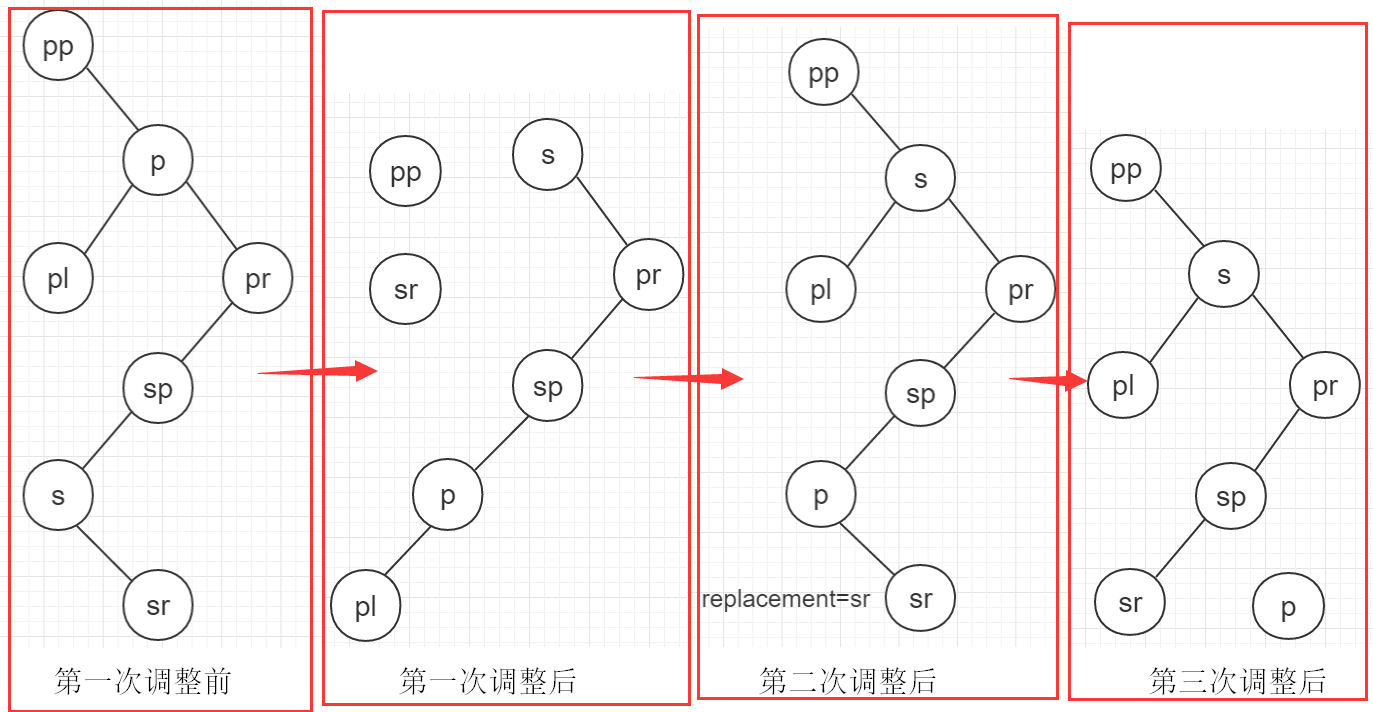# 死循环问题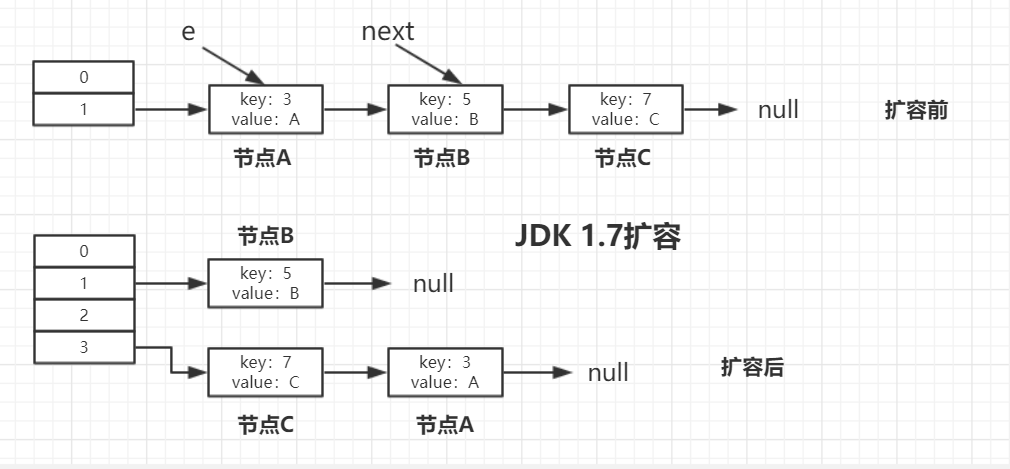# JDK 1.8扩容过程

JDK1.8 普通链表的扩容代码，如下图所示，在上文已经分析过了：主要是在一个do/while中处理同一个位置的所有节点。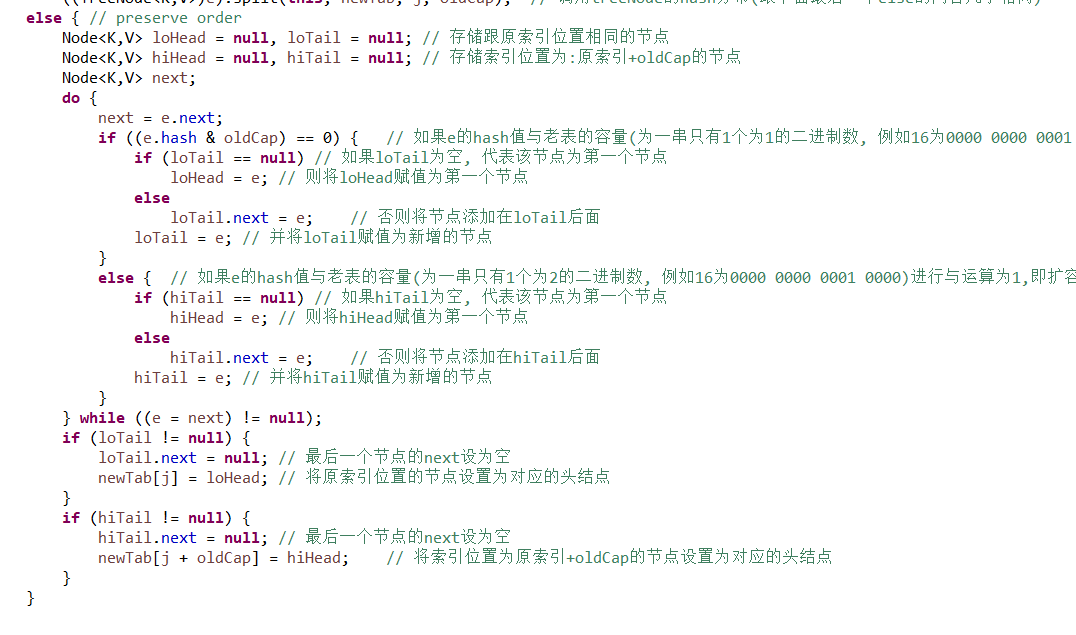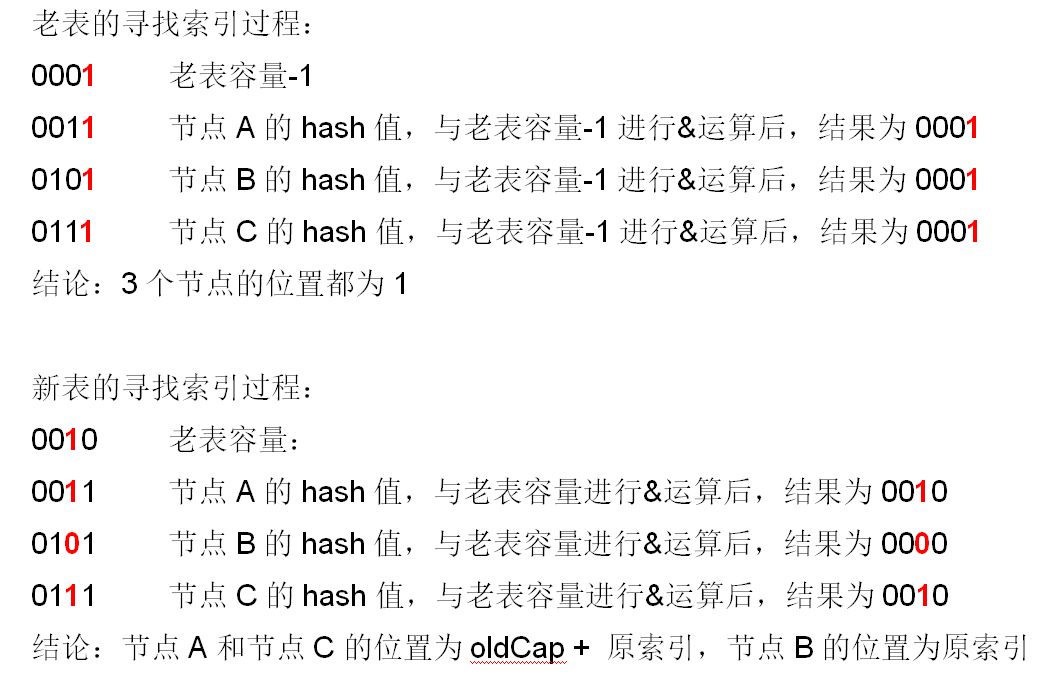## 具体扩容过程：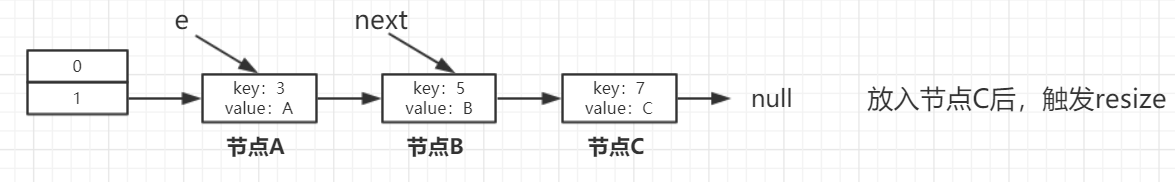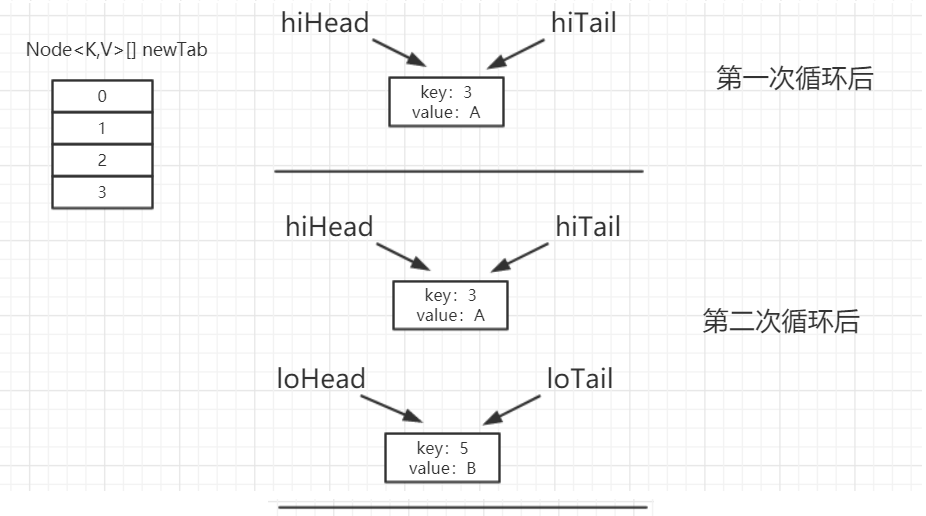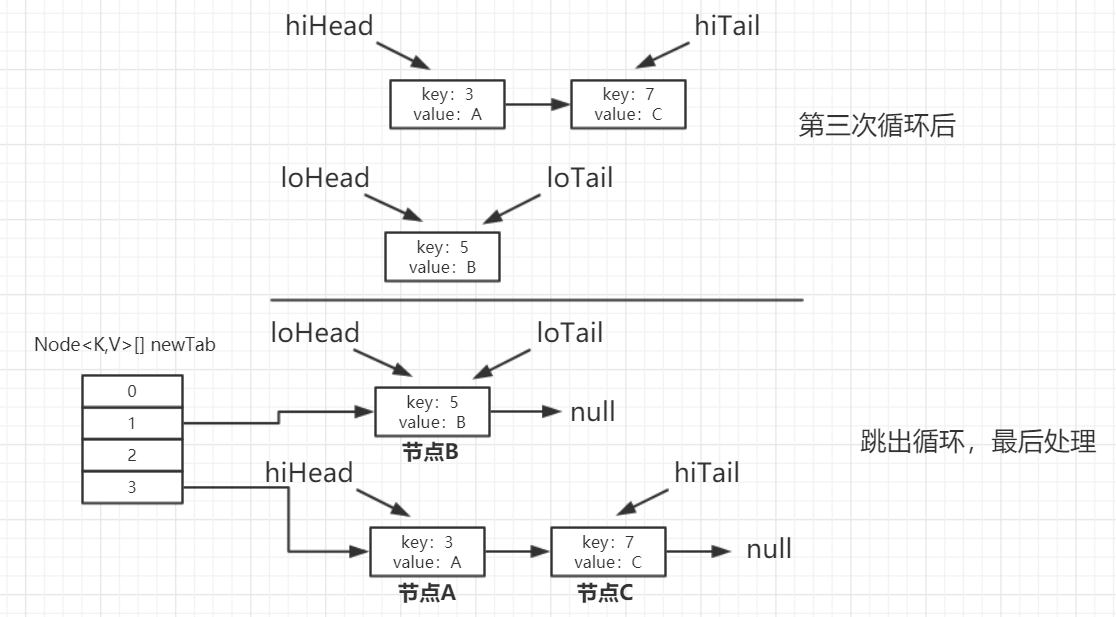# HashMap和Hashtable的区别：

1. HashMap允许key和value为null，Hashtable不允许。
2. HashMap的默认初始容量为16，Hashtable为11。
3. HashMap的扩容为原来的2倍，Hashtable的扩容为原来的2倍加1。
4. HashMap是非线程安全的，Hashtable是线程安全的。
5. HashMap的hash值重新计算过，Hashtable直接使用hashCode。
6. HashMap去掉了Hashtable中的contains方法。
7. HashMap继承自AbstractMap类，Hashtable继承自Dictionary类。

# 总结：

1. HashMap的底层是个Node数组（Node<K,V>[] table），在数组的具体索引位置，如果存在多个节点，则可能是以链表或红黑树的形式存在。
2. 增加、删除、查找键值对时，定位到哈希桶数组的位置是很关键的一步，源码中是通过下面3个操作来完成这一步：1）拿到key的hashCode值；2）将hashCode的高位参与运算，重新计算hash值；3）将计算出来的hash值与(table.length – 1)进行&运算。
4. HashMap在触发扩容后，阈值会变为原来的2倍，并且会进行重hash，重hash后索引位置index的节点的新分布位置最多只有两个：原索引位置或原索引+oldCap位置。例如capacity为16，索引位置5的节点扩容后，只可能分布在新报索引位置5和索引位置21（5+16）。
5. 导致HashMap扩容后，同一个索引位置的节点重hash最多分布在两个位置的根本原因是：1）table的长度始终为2的n次方；2）索引位置的计算方法为“(table.length – 1) & hash”。HashMap扩容是一个比较耗时的操作，定义HashMap时尽量给个接近的初始容量值。
7. 当同一个索引位置的节点在增加后达到9个时，并且此时数组的长度大于等于64，则会触发链表节点（Node）转红黑树节点（TreeNode，间接继承Node），转成红黑树节点后，其实链表的结构还存在，通过next属性维持。链表节点转红黑树节点的具体方法为源码中的treeifyBin(Node<K,V>[] tab, int hash)方法。而如果数组长度小于64，则不会触发链表转红黑树，而是会进行扩容。
8. 当同一个索引位置的节点在移除后达到6个时，并且该索引位置的节点为红黑树节点，会触发红黑树节点转链表节点。红黑树节点转链表节点的具体方法为源码中的untreeify(HashMap<K,V> map)方法。
9. HashMap在JDK1.8之后不再有死循环的问题，JDK1.8之前存在死循环的根本原因是在扩容后同一索引位置的节点顺序会反掉。
10. HashMap是非线程安全的，在并发场景下使用ConcurrentHashMap来代替。

# 参考

HashMap源码（JDK 1.8）